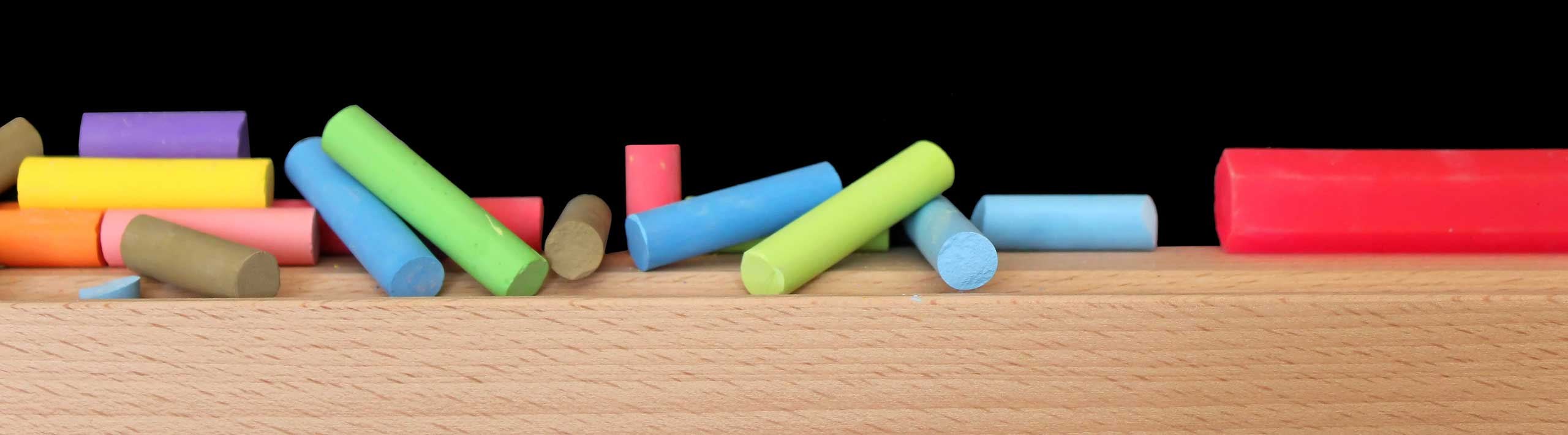# Step by Step Maths Worksheets Solvers

Step by step Maths worksheets solvers to help you create as many questions as you wish and solve them. The interactivity in these worksheets helps you learn how to solve challenging maths problems and learn basic and advanced maths skills. Also the interactivity and graphical interpretation of the solutions in these worksheets, helps you deepen your understanding of the skills needed to solve maths problems. The explanations included in the steps leading to the solution of a given problem are detailed.

If you are using Chrome, you may easily save the solution to any problem generated, in pdf format and use it later (click on the settings button and click Print, then save as pdf). For better experience with these worksheets, use Chrome, Safari or FireFox.

## Functions

Solver to Analyze and Graph a Quadratic Function.

Solver to Analyze and Graph a Linear Function.

Solver to Analyze and Graph a Rational Function.

## Inverse Functions

Step by Step Solver Calculator to Find the Inverse of a Linear Function.

Find the Inverse of a Square Root Function.

Step by Step Solver Calculator to Complete the Square.

Find the Inverse of a Cubic Function.

Find the Inverse of a Rational Function.

## Polynomials

Multiply and Simplify Monomials.

Factor a Polynomial Using Rational Root and Factor theorems.

Step by Step Solver to Find a Polynomial Given its Zeros and a Point.

Step by Step Solver to Factor a Cubic Polynomial Given one of its Zeros.

Find a Cubic Polynomial Passing Through Four Points.

## Domain and Range of Functions

Step by Step Solver to Find the Domain of the Square Root of a Linear Function.

Find the Domain of the Square Root of a Quadratic Function.

## Equations in One Variable

Solve an Equation with a Radical.

Solve an Equation With Two Radicals.

Solve an Equation With Absolute Value.

Solve Rational Equations.

## Equations of Lines

Find the Slope and the y-intercept of a Line.

Find the Points of Intersection of Two Lines.

Step by Step Solver to Find The Equation of a Line Through two Points.

Step by Step Solver to Find The Equation of the Line parallel to Another Line.

Step by Step Solver to Find The Equation of the Line Perpendicular to Another Line.

## Angles in Standard Position

Find Coterminal Angle to a Given Angle.

Find the Reference Angle to a Given Angle.

## Circles

Step by Step Solver Calculator to Find the Points of Intersection of Two Circles.

Step by Step Solver Calculator to Find the Points of Intersection of a Circle and a Line.

## Conics

Find Equation of Parabola Passing Through three Points - Step by Step Solver.

## 3D Lines and Planes

Find The Equation of a Line Through a Point and in the Direction of a Vector in 3D.

Find The Equation of a Line Through two Points in 3D.

Equation of a Plane Through a Point and Parallel to a Vector.

Equation of a Plane Through Three Points.

## Vectors

Find The Cross Product of Vectors.

Find The Angle Between 3D Vectors.

Find the Unit Vector in the Same Direction as a Given Vector.

Find the Unit Vector in the Opposite Direction of a Given Vector.

Find the Magnitude, Direction and Polar Form of a Vector.

Find the Angle Between two Vectors.

## Matrices

Solver Calculator to Find the Inverse of a 3 by 3 Matrix.

Step by Step Solver to Calculate the Determinant of a 3 by 3 Matrix.

## Systems of Equations

Gaussian Elimination Solver Calculator for a 3 by 3 Systems of Equations.

Solver Calculator to Solve 3 by 3 Systems of Equations Using Cramers' Rules.

Algebra Questions and problems
Worksheets with Solutions to Practice For Algebra, College Algebra and Trigonometry